# 8.2 - One Sample Mean

8.2 - One Sample Mean

One sample mean tests are covered in Section 6.2 of the Lock5 textbook.

Concerning one sample mean, the Central Limit Theorem states that if the sample size is large, then the distribution of sample means will be approximately normally distributed with a standard deviation (i.e., standard error) equal to $$\frac{\sigma}{\sqrt n}$$. In this course, a "large" sample size will be defined as one where $$n \ge 30$$.

When constructing confidence interval and conducting hypothesis tests we often do not know the value of $$\sigma$$. In those cases, $$\sigma$$ may be estimated using the sample standard deviation ($$s$$). When we are using $$s$$ to estimate $$\sigma$$ our sampling distribution will not follow a $$z$$ distribution exactly.  Instead, we use what is known as the $$t$$ distribution.  Like the $$z$$ distribution, the $$t$$ distribution is symmetrical. The difference is that its height varies depending on the sample size. By doing so, the distribution becomes more conservative for smaller sample sizes to account for some error that may occur from estimating $$\sigma$$ with $$s$$ from a small sample. As $$n$$ approaches infinity ($$\infty$$) the $$t$$ distribution approaches the standard normal distribution. The next page compares the $$z$$ and $$t$$ distributions.

When constructing confidence intervals and conducting hypothesis tests we will usually be using the $$t$$ distribution when working with one mean. The only exception would be in cases where $$\sigma$$ is known. This scenario is most common in the fields of education and psychology where some tests are normed to have a certain $$\mu$$ and $$\sigma$$. In those cases, the $$z$$ distribution can be used.

In terms of language, all of these tests could be called "single sample mean tests" or "one sample mean tests."  We could also specify the sampling distribution by using the term "single sample mean $$t$$ test" or "single sample mean $$z$$ test."

The flow chart below may help you in determining which method should be used when constructing a sampling distribution for one sample mean.

#### One Sample Mean

Identify when z and t distributions should be used.

• Is the population known to be normally distributed?
• Is the population standard deviation known?
• Is the sample size at least 30?

# 8.2.1 - t Distribution

8.2.1 - t Distribution

The height of the t distribution is determined by the number of degrees of freedom (df). For a one sample mean test, $$df=n-1$$.

The first plot below compares the standard normal distribution (i.e., z distribution) to a t distribution. The solid blue line is the standard normal distribution and the dashed red line is a t distribution with 2 degrees of freedom. Here, the tails of the t distribution are higher than the tails of the normal distribution.If you think about the area under the curve, the higher tails mean that more area will fall in the tails. For example, as seen in the following two plots, $$P(z>2.00)=0.0227501$$ while $$P(t_{df=2}>2.00)=0.0917517$$.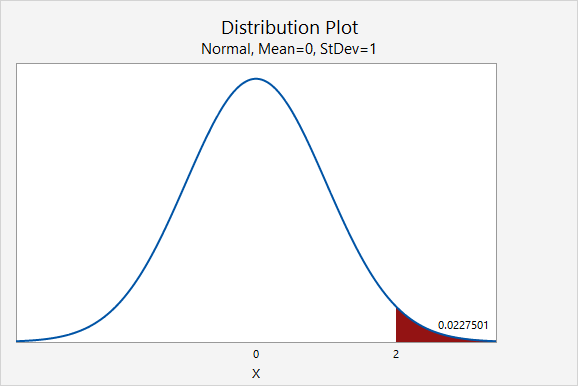The next plot compares the standard normal distribution to a t distribution with 10 degrees of freedom. Notice that the two distributions are becoming more similar as the sample size increases.The next plot compares the standard normal distribution to a t distribution with 30 degrees of freedom.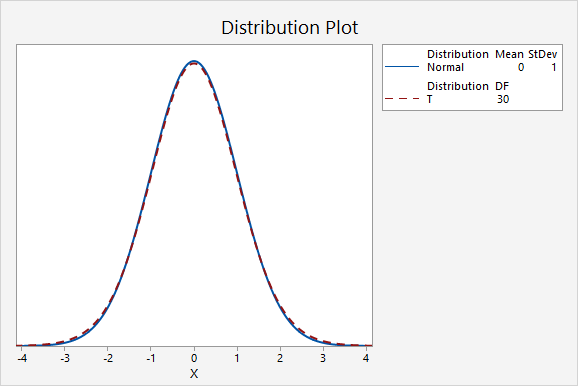In the final graph, the standard normal distribution is compared to a t distribution with 500 degrees of freedom. Here, the two distributions are nearly identical. As the degrees of freedom approach infinity, the t distribution approaches (i.e., becomes more similar to) the standard normal distribution.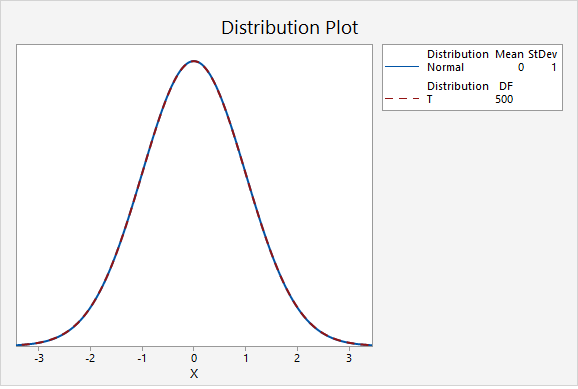## Minitab®

The procedures for constructing t distributions in Minitab are similar to those for constructing z distributions. We can construct a probability distribution plot to find the t* multiplier when constructing a confidence interval. And, we can construct a plot to find the p-value when conducting a hypothesis test.

#### Steps for finding the t* multiplier

1. In Minitab, select Graph > Probability Distribution Plot > View Probability
2. Change the Distribution to t
3. Enter your Degrees of freedom
4. Select Options
5. Choose A specified probability
6. Select Equal tails
7. For Probability enter the value that is split between the two tails (e.g., for a 90% confidence interval you would enter 0.10)

#### Steps for finding the p value given a t test statistic

1. In Minitab, select Graph > Probability Distribution Plot > View Probability
2. Change the Distribution to t
3. Enter your Degrees of freedom
4. Select A specified x value
5. Select Right tail, Left tail, or Equal tails, depending on the direction of your alternative hypothesis
6. For X value enter the t test statistic

# 8.2.2 - Confidence Intervals

8.2.2 - Confidence Intervals

Confidence intervals are used to estimate unknown population parameters. Because the population standard deviation ($$\sigma$$) will almost always be unknown in situations in which we are constructing confidence intervals for means, the $$t$$ distribution is used to estimate the sampling distribution. The following pages will show you how to construct a confidence interval for a population mean using formulas and using Minitab. Similar to how we computed necessary minimum sample sizes for confidence intervals for proportions, we will also compute the necessary minimum sample size for constructing a confidence interval for a mean.

# 8.2.2.1 - Formulas

8.2.2.1 - Formulas

Earlier in this lesson we considered confidence intervals for proportions and the multiplier in our intervals was a value from the standard normal (i.e., $$z$$) distribution. But, what if our variable of interest is a quantitative variable and we want to estimate a population mean?

We apply similar techniques when constructing a confidence interval for a mean, but now we are interested in estimating the population mean ($$\mu$$) by using the sample statistic ($$\overline{x}$$) and the multiplier is a $$t$$ value. Similar to the $$z$$ values that you used as the multiplier for constructing confidence intervals for population proportions, here you will use $$t$$ values as the multipliers. Because $$t$$ values vary depending on the number of degrees of freedom (df), you will need to use statistical software to look up the appropriate $$t$$ value for each confidence interval that you construct. The degrees of freedom will be based on the sample size. Since we are working with one sample here, $$df=n-1$$.

## Minitab® – Finding t* Multipliers

To find the t* multiplier for a 98% confidence interval with 15 degrees of freedom:

1. In Minitab, select Graph > Probability Distribution Plot > View Probability
2. Change the Distribution to t
3. Enter 15 for the Degrees of freedom
4. Select Options
5. Choose A specified probability
6. Select Equal tails
7. For Probability enter 0.02 (if there is 0.98 in the middle, then 0.02 is split equally between the left and right tails)

This should result in an output similar to the output below. Note that your results may be slightly different due to random sampling variation.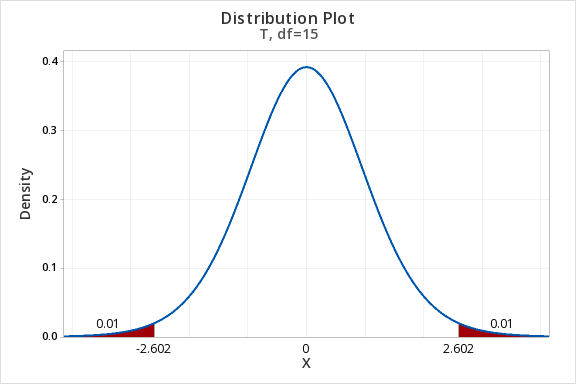Let’s review some of symbols and equations that we learned in previous lessons:

Sample size $$n$$ $$\mu=\frac{\sum X}{N}$$ $$\overline{x}= \frac{\sum x}{n}$$ $$SE=\frac{s}{\sqrt{n}}$$ $$t^{*}$$ $$df=n-1$$

Recall the general form for a confidence interval:

General Form of Confidence Interval
$$sample\ statistic\pm\underbrace{(multiplier)\ (standard\ error)}_{\textbf{margin of error}}$$

When constructing a confidence interval for a population mean the point estimate is the sample mean, $$\overline{x}$$. The multiplier is taken from a $$t$$ distribution. And, the standard error is equal to $$\frac{s}{\sqrt{n}}$$.

Confidence Interval for a Population Mean
$$\underbrace{\overline{x}}_{\text{sample statistic}} \pm \overbrace{t^{*}}^{\text{multiplier}} \underbrace{ \dfrac{s}{\sqrt{n}}}_{\text{standard error}}$$

On the following pages we will walk through examples of constructing confidence intervals for population means by hand. Then, you will learn how to compute confidence intervals using Minitab.

# 8.2.2.1.1 - Example: MLB Age

8.2.2.1.1 - Example: MLB Age

In a sample of 30 current MLB pitchers, the mean age was 28 years with a standard deviation of 4.4 years. Construct a 95% confidence interval to estimate the mean age of all current MLB pitchers.

This is what we know: $$n=30$$, $$\overline{x}=28$$, and $$s=4.4$$.

In order to compute the confidence interval for $$\mu$$ we will need the t multiplier and the standard error ($$\frac{s}{\sqrt{n}}$$).

$$df=n-1=30-1=29$$For a 95% confidence interval with 29 degrees of freedom, $$t^{*}=2.045$$

$$SE=\dfrac{s}{\sqrt{n}}=\dfrac{4.4}{\sqrt{30}}=0.803$$

Thus, our confidence interval for $$\mu$$ is: $$28\pm 2.045(0.803)=28\pm1.643=[26.357,29.643]$$

We are 95% confident that the population mean age is between 26.357 and 29.643.

# 8.2.2.1.2- Example: Sleep Deprivation

8.2.2.1.2- Example: Sleep Deprivation

In a class survey, students were asked how many hours they sleep per night. In the sample of 22 students, the mean was 5.77 hours with a standard deviation of 1.572 hours. That distribution was approximately normal. Let’s construct a 95% confidence interval for the mean number of hours slept per night in the population from which this sample was drawn.

This is what we know: $$n=22$$, $$\overline{x}=5.77$$, and $$s=1.572$$.

In order to compute the confidence interval for $$\mu$$ we will need the t multiplier and the standard error ($$\frac{s}{\sqrt{n}}$$).

$$df=n-1=22-1=21$$For a 95% confidence interval with 21 degrees of freedom, $$t^{*}=2.080$$

$$SE=\frac{s}{\sqrt{n}}=\frac{1.572}{\sqrt{22}}=0.335$$

Thus, our confidence interval for $$\mu$$ is: $$5.77\pm 2.080(0.335)=5.77\pm0.697=[5.073,\;6.467]$$

We are 95% confident that the population mean is between 5.073 and 6.467 hours.

What if we wanted to be more conservative and construct a 99% confidence interval?

The only thing that would change is our multiplier. Now, $$t^{*}=2.831$$.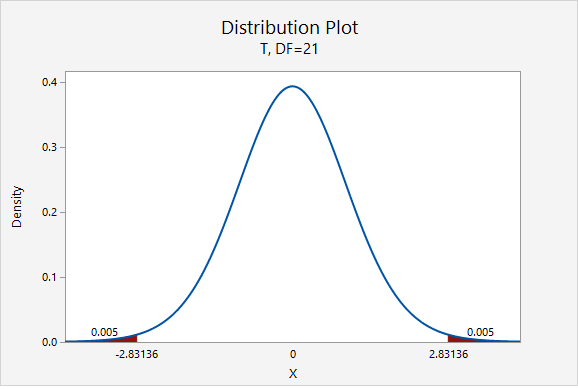$$5.77\pm 2.831(0.335)=5.77\pm0.948=[4.822,\;6.718]$$

We are 99% confident that the population mean is between 4.822 and 6.718 hours.

# 8.2.2.1.3 - Example: Milk

8.2.2.1.3 - Example: Milk

A study of 66,831 dairy cows found that the mean milk yield was 12.5 kg per milking with a standard deviation of 4.3 kg per milking (data from Berry, et al., 2013). Construct a 95% confidence interval for the average milk yield in the population.

First, let's compute the standard error:

$$SE=\dfrac{s}{\sqrt{n}}=\dfrac{4.3}{\sqrt{66831}}=0.0166$$

The standard error is small because the sample size is very large.

Next, let's find the $$t^*$$ multiplier:

$$df=66831-1=66830$$$$t^{*}=1.960$$

Now, we can construct our 95% confidence interval:

95% C.I.: $$12.5\pm1.960(0.017)=12.5\pm0.033=[12.467,\;12.533]$$

We are 95% confident that the mean milk yield in the population is between 12.467 and 12.533 kg per milking.

# 8.2.2.2 - Minitab: Confidence Interval of a Mean

8.2.2.2 - Minitab: Confidence Interval of a Mean

Here you will learn how to use Minitab to construct a confidence interval for a mean. The procedure is similar to the one that you learned earlier in this lesson for constructing a confidence interval for a proportion. The following example walks through this procedure when data are in a Minitab work. At the bottom of this page you will find instructions for using Minitab with summarized data.

## Minitab® – Confidence Interval for a Mean

To create a 95% confidence interval of mean height in Minitab:

1. Open the data set: fall2016stdata.csv
2. In Minitab, select Stat > Basic Statistics > 1-sample t
3. In this case we have our data in the Minitab worksheet so we will use the default One or more samples, each in a column
4. Double click the variable Height in the box on the left to insert the variable into the box
5. Select Options
6. The default Confidence level is 95
7. Click OK and OK

This should result in the following output:

#### Descriptive Statistics

N Mean StDev SE Mean 95% CI for $$\mu$$
525 67.009 4.462 0.195 (66.627, 67.392)

$$\mu$$: mean of Height

## What if we have summarized data and not data in a Minitab worksheet?

If you do not have a Minitab worksheet filled with data concerning individuals, but instead have summarized data (e.g., the values of $$s$$, $$\overline{x}$$, and $$n$$), you would skip step 1 above and in step 3 you would select Summarized data

# 8.2.2.2.1 - Example: Age of Pitchers (Summarized Data)

8.2.2.2.1 - Example: Age of Pitchers (Summarized Data)

## Example: Estimating the average MLB Pitcher's age

In a sample of 30 current MLB pitchers, the mean age was 28 years with a standard deviation of 4.4 years. Construct a 95% confidence interval to estimate the mean age of all current MLB pitchers.

We know that n = 30, $$\bar{x}=28$$, and s = 4.4.

To create a 95% confidence interval of mean age in Minitab:

1. In Minitab, select Stat > Basic Statistics > 1-sample t
2. In this case we have summarized data so select Summarized Data from the dropdown
3. Enter 30 for the sample size, 28 for the sample mean and 4.4 for the standard deviation.
4. Select Options
5. The default Confidence level is 95
6. Click OK and OK

This should result in the following output:

#### Descriptive Statistics

N Mean StDev SE Mean 95% CI for $$\mu$$
30 28.000 4.400 0.803 (26.357, 29.643)

$$\mu$$: population mean of sample

We are 95% confident that the population mean age is between 26.357 and 29.643 years.

# 8.2.2.2.2 - Example: Coffee Sales (Data in Column)

8.2.2.2.2 - Example: Coffee Sales (Data in Column)

For 48 days data concerning sales were collected from one student-run cafe. Let's construct a 95% confidence interval for the mean number of coffees sold per day.

To create a 95% confidence interval of mean number of coffees sold per day in Minitab:

1. Open the file: cafedata.mpx
2. In Minitab, select Stat > Basic Statistics > 1-sample t
3. In this case the data is in a worksheet so select use One or more samples, each in a column
4. Select the variable Coffees
5. Select Options
6. The default Confidence level is 95
7. Click OK and OK

This should result in the following output:

#### Descriptive Statistics

N Mean StDev SE Mean 95% CI for $$\mu$$
47 21.51 11.08 1.62 (18.26, 24.76)

$$\mu$$: population mean of Coffees

We are 95% confident that the population mean number of coffees solder per day is between 18.26 and 24.76.

# 8.2.2.3 - Computing Necessary Sample Size

8.2.2.3 - Computing Necessary Sample Size

Calculating the sample size necessary for estimating a population mean with a given margin of error and level of confidence is similar to that for estimating a population proportion. However, since the $$t$$ distribution is not as “neat” as the standard normal distribution, the process can be iterative. (Recall, the shape of the $$t$$ distribution is different for each degree of freedom). This means that we would solve, reset, solve, reset, etc. until we reached a conclusion. Yet, we can avoid this iterative process if we employ an approximate method based on $$t$$ distribution approaching the standard normal distribution as the sample size increases. This approximate method invokes the following formula:

Finding the Sample Size for Estimating a Population Mean
$$n=\dfrac{z^{2}\widetilde{\sigma}^{2}}{M^{2}}=\left ( \dfrac{z\widetilde{\sigma}}{M} \right )^2$$

$$z$$ = z multiplier for given confidence level
$$\widetilde{\sigma}$$ = estimated population standard deviation
$$M$$ = margin of error

The sample standard deviation may be estimated on the basis of prior research studies.

# 8.2.2.3.1 - Example: Estimating IQ

8.2.2.3.1 - Example: Estimating IQ

## Example: Estimating IQ

A team of researchers wants to estimate the mean IQ of students enrolled at one prestigious university. Previous research studies have examined samples of students from other similar universities and usually find results around $$\overline{x}=120$$ and $$s=10$$. In order to construct a 90% confidence interval with a margin of error of $$\pm2$$  IQ points, what sample size should be obtained?

As shown in the probability distribution plot below, the z value associated with a 90% confidence interval is 1.645.The estimated standard deviation is given to be 10 and the desired margin of error is given to be 2.

$$n=\dfrac{z^{2}\widetilde{\sigma}^{2}}{M^{2}}=\dfrac{1.645^{2}(10^{2})}{2^{2}}=67.615$$

We round up to 68. The research team should attempt to obtain a sample of at least 68 individuals.

# 8.2.2.3.2 - Video Example: Age

8.2.2.3.2 - Video Example: Age

# 8.2.2.3.3 - Video Example: Cookie Weights

8.2.2.3.3 - Video Example: Cookie Weights

# 8.2.3 - Hypothesis Testing

8.2.3 - Hypothesis Testing

In this section we will be comparing one sample mean to one known or hypothesized population value. In Lesson 5 you learned how to conduct randomization tests. Here, you will learn how to conduct a one sample mean $$t$$ test and a one sample mean $$z$$ test. The $$t$$ distribution is used to estimate the sampling distribution when the sample size is large (at least 30) or when the population is known to be normally distributed (but $$\sigma$$ is unknown). The $$z$$ distribution is used on rare occasions when the population is normal and the population standard deviation is known. Note that for this course the one sample mean $$z$$ test is optional; it used only in specific cases where the population is known to be normally distributed and when the population standard deviation ($$\sigma$$) is known. The most commonly used one sample mean test is the "one sample mean $$t$$ test" which is also known as a "single sample mean $$t$$ test."

# 8.2.3.1 - One Sample Mean t Test, Formulas

8.2.3.1 - One Sample Mean t Test, Formulas

## Five Step Hypothesis Testing Procedure

1. Check assumptions and write hypotheses

Data must be quantitative. In order to use the t distribution to approximate the sampling distribution either the sample size must be large ($$\ge\ 30$$) or the population must be known to be normally distributed. The possible combinations of null and alternative hypotheses are:

Research Question Is the mean different from $$\mu_{0}$$? Is the mean greater than $$\mu_{0}$$? Is the mean less than $$\mu_{0}$$?
Null Hypothesis, $$H_{0}$$ $$\mu=\mu_{0}$$ $$\mu=\mu_{0}$$ $$\mu=\mu_{0}$$
Alternative Hypothesis, $$H_{a}$$ $$\mu\neq \mu_{0}$$ $$\mu> \mu_{0}$$ $$\mu<\mu_{0}$$
Type of Hypothesis Test Two-tailed, non-directional Right-tailed, directional Left-tailed, directional

where $$\mu_{0}$$ is the hypothesized population mean.

2. Calculate the test statistic

For the test of one group mean we will be using a $$t$$ test statistic:

Test Statistic: One Group Mean

$$t=\dfrac{\overline{x}-\mu_0}{\frac{s}{\sqrt{n}}}$$

$$\overline{x}$$ = sample mean
$$\mu_{0}$$ = hypothesized population mean
$$s$$ = sample standard deviation
$$n$$ = sample size

Note that structure of this formula is similar to the general formula for a test statistic:

$$\dfrac{sample\;statistic-null\;value}{standard\;error}$$

3. Determine the p-value

When testing hypotheses about a mean or mean difference, a $$t$$ distribution is used to find the $$p$$-value. These $$t$$ distributions are indexed by a quantity called degrees of freedom, calculated as $$df = n – 1$$ for the situation involving a test of one mean or test of mean difference. The $$p$$-value can be found using Minitab.

4. Make a decision

If $$p \leq \alpha$$ reject the null hypothesis.

If $$p>\alpha$$ fail to reject the null hypothesis.

5. State a "real world" conclusion

Based on your decision in Step 4, write a conclusion in terms of the original research question.

The new few pages will walk you through examples before giving you the opportunity to do two on your own.

# 8.2.3.1.1 - Video Example: Book Costs

8.2.3.1.1 - Video Example: Book Costs

Research question: Does the average Penn State student spend more than \$300 each semester on textbooks? In a sample of 226 Penn State students, the mean cost of a student’s textbooks was \$344 with a standard deviation of \$106. # 8.2.3.1.2 : Example: Pulse Rate 8.2.3.1.2 : Example: Pulse Rate A research study measured the pulse rates of 57 college men and found a mean pulse rate of 70.4211 beats per minute with a standard deviation of 9.9480 beats per minute. Researchers want to know if the mean pulse rate for all college men is different from the current standard of 72 beats per minute. 1. Check assumptions and write hypotheses Pulse rates are quantitative. The sampling distribution will be approximately normally distributed because $$n \ge 30$$. This is a two-tailed test because we want to know if the mean pulse rate is different from 72. $$H_{0}:\mu=72$$ $$H_{a}: \mu\neq 72$$ 2. Calculate the test statistic Test Statistic: One Group Mean $$t=\dfrac{\overline{x}-\mu_0}{\dfrac{s}{\sqrt{n}}}$$ $$\overline{x}$$ = sample mean $$\mu_{0}$$ = hypothesized population mean $$s$$ = sample standard deviation $$n$$ = sample size $$t=\dfrac{\overline{x}-\mu_0}{\dfrac{s}{\sqrt{n}}}=\dfrac{70.4211-72}{\dfrac{9.9480}{\sqrt{57}}}=-1.198$$ Our $$t$$ test statistic is -1.198 3. Determine the p-value $$df=n-1=57-1=56$$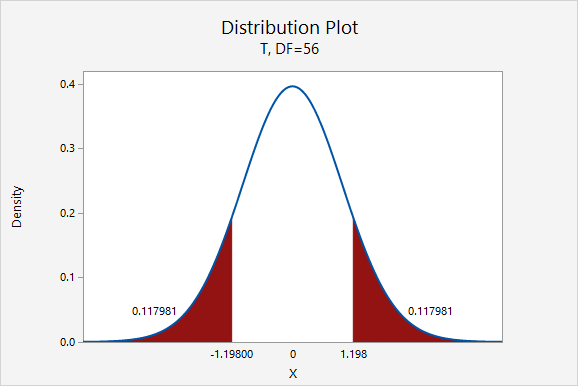$$p=0.117981+0.117981=0.235962$$ Given that the null hypothesis is true and $$\mu=72$$, the probability of taking a random sample of $$n=57$$ and finding a sample mean this or more extremely different is 0.235962. This is our p-value. 4. Make a decision $$p>.05$$, therefore we fail to reject the null hypothesis. 5. State a "real world" conclusion There is not sufficient evidence to state that the mean pulse of college men is different from 72. # 8.2.3.1.3 - Example: Coffee 8.2.3.1.3 - Example: Coffee In the population of Americans who drink coffee, the average daily consumption is 3 cups per day. A university wants to know if their students tend to drink more coffee than the national average. They ask a random sample of 50 students how many cups of coffee they drink each day and found $$\overline{x}=3.8$$ and $$s=1.5$$. Do they have evidence that their students drink more than the national average? 1. Check assumption and write hypotheses Amount of coffee consumed is a quantitative variable. We are given that random sampling methods were employed. Because $$n \ge 30$$, we can approximate the sampling distribution using a t distribution. This is a right-tailed test because we want to know if the mean in the sample is greater than the national average. $$H_{0}:\mu= 3$$ $$H_{a}:\mu>3$$ 2. Calculate the test statistic Test Statistic: One Group Mean $$t=\dfrac{\overline{x}-\mu_0}{\dfrac{s}{\sqrt{n}}}$$ $$\overline{x}$$ = sample mean $$\mu_{0}$$ = hypothesized population mean $$s$$ = sample standard deviation $$n$$ = sample size $$t=\dfrac{\overline{x}-\mu_0}{\dfrac{s}{\sqrt{n}}}=\dfrac{3.8-3}{\dfrac{1.5}{\sqrt{50}}}=3.771$$ Our $$t$$ test statistic is 3.771 3. Determine the p-value $$df=n-1=50-1=49$$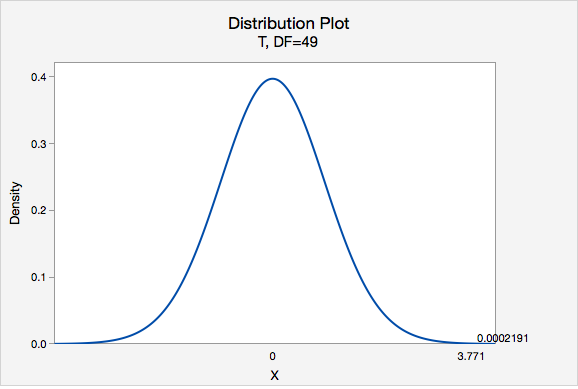Using Minitab, we can find that $$P(t > 3.771) =0.0002191$$ p-value = 0.0002191 If $$\mu=3$$, then the probability of taking a random sample of $$n=50$$ and finding $$\overline{x} \geq 3$$ is 0.0002191 4. Make a decision $$p\leq.05$$, therefore we reject the null hypothesis. 5. State a "real world" conclusion There is evidence to state the mean number of coffees consumed in the population of all students at this university is greater than 3. # 8.2.3.1.4 - Example: Transportation Costs 8.2.3.1.4 - Example: Transportation Costs According to CNN, in 2011, the average American spent \$16,803 on housing. A suburban community wants to know if their residents spent less than this national average. In a survey of 30 randomly selected residents, they found that they spent an annual average of \$15,800 with a standard deviation of \$2,600.

1. Check assumptions and write hypotheses

Housing costs are quantitative. Because $$n \ge 30$$, the sampling distribution can be approximated using the $$t$$ distribution.

This is a left-tailed test because we want to know if residents of this community spent less than the national average.

$$H_{0}:\mu=16803$$
$$H_{a}:\mu<16803$$

2. Calculate the test statistic
Test Statistic: One Group Mean

$$t=\dfrac{\overline{x}-\mu_0}{\dfrac{s}{\sqrt{n}}}$$

$$\overline{x}$$ = sample mean
$$\mu_{0}$$ = hypothesized population mean
$$s$$ = sample standard deviation
$$n$$ = sample size

$$t=\dfrac{\overline{x}-\mu_0}{\dfrac{s}{\sqrt{n}}}=\dfrac{15800-16803}{\dfrac{2600}{\sqrt{30}}}=-2.113$$

Our t test statistics is -2.113

3. Determine the p-value

$$df=n-1=30-1=29$$

This is a left-tailed test so we want to know the probability of $$t < -2.113$$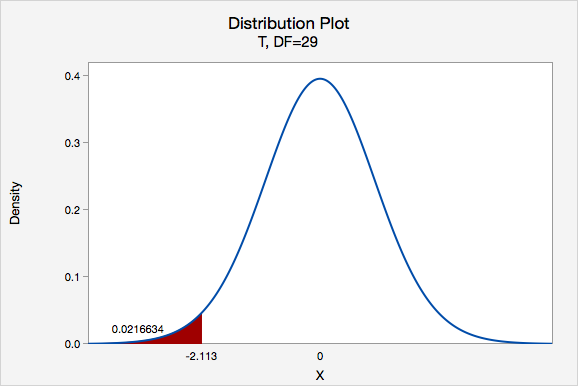Using Minitab we can find that $$p=0.0216634$$

4. Make a decision

$$p\leq .05$$, therefore we reject the null hypothesis.

5. State a "real world" conclusion

There is evidence to state that on average residents of this community spent less than the national average on housing in 2011.

# 8.2.3.2 - Minitab: One Sample Mean t Tests

8.2.3.2 - Minitab: One Sample Mean t Tests

A hypothesis test for one group mean can be conducted in Minitab using raw data or summarized data.

• If you have a data file with every individual's observation, then you have raw data
• If you do not have each individual's observation, but rather have the sample mean, sample standard deviation, and sample size, then you have summarized data

The next two pages will show you how to use Minitab to conduct a one-sample mean t-test using either raw data or summarized data. There is also one example of using Minitab to conduct a one-sample mean z test which is only performed if the population is known to be normally distributed and the population standard deviation ($$\sigma$$) is available.

# 8.2.3.2.1 - Minitab: 1 Sample Mean t Test, Raw Data

8.2.3.2.1 - Minitab: 1 Sample Mean t Test, Raw Data

## Minitab® – One Sample Mean t Test Using Raw Data

Research question: Is the mean GPA in the population different from 3.0?

• Null hypothesis: $$\mu$$ = 3.0
• Alternative hypothesis: $$\mu$$ ≠ 3.0

The GPAs of 226 students are available.

A one sample mean $$t$$ test should be performed because the shape of the population is unknown, however the sample size is large ($$n$$ ≥ 30).

To perform a one sample mean $$t$$ test in Minitab using raw data:

1. Open the Minitab file: class_survey.mpx
2. Select Stat > Basic Statistics > 1-sample t
3. Select One or more samples, each in a column from the dropdown
4. Double-click on the variable GPA to insert it into the Sample box
5. Check the box Perform a hypothesis test
6. For the Hypothesized mean enter 3
7. Select Options
8. Use the default Alternative hypothesis of Mean ≠ hypothesized value
9. Use the default Confidence level of 95
10. Click OK and OK

This should result in the following output:

N Mean StDev SE Mean 95% CI for $$\mu$$
226 3.2311 0.5104 0.0340 (3.1642, 3.2980)
$$\mu$$: population mean of GPA
Null hypothesis H0: $$\mu$$ = 3 H1: $$\mu$$ ≠ 3
T-Value P-Value
6.81 0.000

## Summary of Results

We could summarize these results using the five step hypothesis testing procedure:

1. Check assumptions and write hypotheses

We do not know if the population is normally distributed, however the sample size is large ($$n \ge 30$$) so we can perform a one sample mean t test.

$$H_0\colon \mu = 3.0$$
$$H_a\colon \mu \ne 3.0$$

2. Calculate the test statistic

$$t (225) = 6.81$$

3. Determine the p-value

$$p < 0.0001$$

4. Make a decision

$$p \le \alpha$$, reject the null hypothesis

5. State a "real world" conclusion

There is evidence that the mean GPA in the population is different from 3.0

# 8.2.3.2.2 - Minitab: 1 Sample Mean t Test, Summarized Data

8.2.3.2.2 - Minitab: 1 Sample Mean t Test, Summarized Data

## Minitab® – One Sample Mean t Test Using Summarized Data

Here we are testing $$H_{a}\colon\mu\neq72$$ and are given $$n=35$$, $$\bar{x}=76.8$$, and $$s=11.62$$.

We do not know the shape of the population, however the sample size is large ($$n \ge 30$$) therefore we can conduct a one sample mean $$t$$ test.

To perform a one sample mean $$t$$ test in Minitab using raw data:

1. In Minitab, select Stat > Basic Statistics > 1-sample t
2. Select Summarized data from the dropdown
3. Enter 35 for the sample size, 76.8 for the sample mean and 11.62 for the standard deviation.
4. Check the box Perform a hypothesis test
5. For the Hypothesized mean enter 72
6. Select Options
7. Use the default Alternative hypothesis of Mean ≠ hypothesized value
8. Use the default Confidence level of 95
9. Click OK and OK

This should result in the following output:

#### Descriptive Statistics

N Mean StDev SE Mean 95% CI for $$\mu$$
35 76.80 11.62 1.96 (72.81, 80.79)
$$\mu$$: population mean of Sample
Null hypothesis H0: $$\mu$$ = 72 H1: $$\mu$$ ≠ 72
T-Value P-Value
2.44 0.0199

We could summarize these results using the five step hypothesis testing procedure:

1. Check assumptions and write hypotheses

The shape of the population distribution is unknown, however with $$n \ge 30$$ we can perform a one sample mean t test.

$$H_0\colon \mu = 72$$
$$H_a\colon \mu \ne 72$$

2. Calculate the test statistic

$$t (34) = 2.44$$

3. Determine the p-value

$$p = 0.0199$$

4. Make a decision

$$p \le \alpha$$, reject the null hypothesis

5. State a "real world" conclusion

There is evidence that the population mean is different from 72.

# 8.2.3.3 - One Sample Mean z Test (Optional)

8.2.3.3 - One Sample Mean z Test (Optional)

A one sample mean $$z$$ test is used when the population is known to be normally distributed and when the population standard deviation ($$\sigma$$) is known. This most frequently occurs in the social sciences when standardized measures are used such as IQ, SAT, ACT, or GRE scores, for which the population parameters are known.

The formula for computing a $$z$$ test statistic for one sample mean is identical to that of computing a $$t$$ test statistic for one sample mean, except now the population standard deviation is known and can be used in computing the standard error.

z Test Statistic: One Group Mean
$$z=\dfrac{\overline{x}-\mu_0}{\dfrac{\sigma}{\sqrt{n}}}$$

$$\overline{x}$$ = sample mean
$$\mu_{0}$$ = hypothesized population mean
$$s$$ = sample standard deviation
$$n$$ = sample size

The other primary difference between the one sample mean $$t$$ test and the one sample mean $$z$$ test is the latter uses the standard normal distribution (i.e., $$z$$ distribution) in determining the $$p$$-value. Below are the directions for conducting a one sample mean $$z$$ test in Minitab.

## Minitab® – Performing a One Sample Mean z Test

Research question: Are the IQ scores of students at one college-prep school above the national average?

Scores on one American IQ test are normed to have a mean of 100 and standard deviation of 15. In a simple random sample of 25 students at this school the mean was 110.

To perform a one-sample mean test in Minitab using summarized data:

1. In Minitab, select Stat > Basic Statistics > 1-sample Z
2. Select Summarized data from the dropdown
3. Enter 25 for the sample size, 110 for the sample mean and 15 for the known standard deviation.
4. Check the box Perform a hypothesis test
5. For the Hypothesized mean enter 100
6. Select Options
7. Use the default Alternative hypothesis of Mean > hypothesized value
8. Use the default Confidence level of 95
9. Click OK and OK

This should result in the following output:

#### Descriptive Statistics

N Mean SE Mean 95% Lower Bound for $$\mu$$
25 110.00 3.00 105.07

$$\mu$$: population mean of Sample
Known standard deviation = 15

#### Test

Null hypothesis H0: $$\mu$$ = 100 H1: $$\mu$$ > 100
Z-Value P-Value
3.33 0.000

## Summary of Results

We could summarize these results using the five step hypothesis testing procedure:

1. Check assumptions and write hypotheses

The population is known to be normally distributed and the population standard deviation is known to be 15. With these two conditions met we can conduct a one sample mean z test

$$H_0\colon \mu = 100$$
$$H_a\colon \mu > 100$$

2. Calculate the test statistic

From the Minitab output, $$z = 3.33$$

3. Determine the p-value

From the Minitab output, $$p = 0.000$$

4. Make a decision

$$p \le \alpha$$, reject the null hypothesis

5. State a "real world" conclusion

There is evidence that the mean IQ score of all students at this school is greater than 100.

  Link ↥ Has Tooltip/Popover Toggleable Visibility